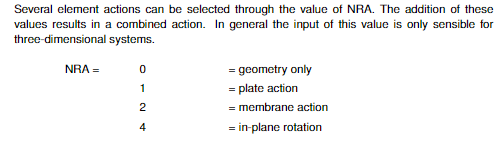# NRA parameter for QUAD element

Hello, I would like a description of what the options for NRA are in the QUAD element. In the SOFIMSHA manual I found this.However, the default option is 7 and when I export the database with the ROTATIONAL stiffness deactivated (which I configured using SOFiPLUS), then the NRA changes to 3. That is an option that I haven’t found in the manuals.

So the question: is there any other parameters for the NRA parameter (5, 6, … ??) and what those parameters mean?

Der Wert von NRA wird bitweise codiert:
kein Bit gesetzt = 0 (0000B)
Bit 1 gesetzt = 1 (0001B)
Bit 2 gesetzt = 2 (0010B)
Bit 3 gesetzt = 4 (0100B)
Bit 1+2 gesetzt = 1+2=3 (0011B)
Bit 1+2+3 gesetzt = 1+2+4=7 (0111B)